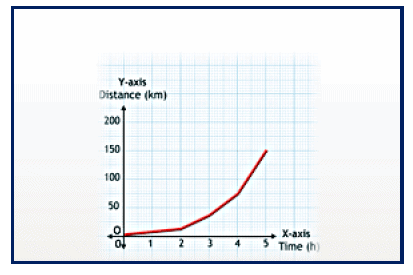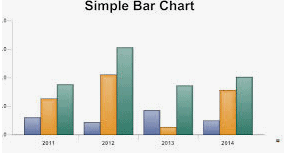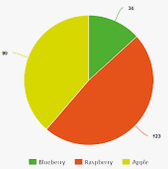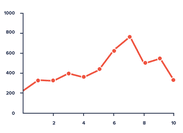Courses

# Chapter Notes 1 - Chapter 13 : Motion and Time, Class 7, Science | EduRev Notes

## Class 7 : Chapter Notes 1 - Chapter 13 : Motion and Time, Class 7, Science | EduRev Notes

The document Chapter Notes 1 - Chapter 13 : Motion and Time, Class 7, Science | EduRev Notes is a part of the Class 7 Course Science Class 7.
All you need of Class 7 at this link: Class 7

Motion and Time

Time and Speed
An object is said to be moving slowly if it covers less distance in a given time.

Moving Slowly
An object is said to be moving slowly if it covers less distance in a given time.

Moving Fast
An object is said to be moving fast if it covers more distance in a given time.

Speed
The distance covered by an object in a unit time is defined as speed. Objects are said to be in fast or slow motion depending upon the speed of their motion. Speed varies from object to object, and it can be animate or inanimate. Speed is calculated using the expression Speed = Distance/Time

The basic unit for time is second, and it can be calculated in minutes and hours, too, depending on the need. The basic unit for distance is metre. So, the unit for speed is metres per second (m/s). Large speeds are measured in kilometre per hour (km/h). The symbols for units are
written in singular form only. For example, the speed of the cheetah is 112 km/h, and Speed of giant tortoise = 0.27 km/h.

Average Speed
The average speed of a moving object is defined as the total distance covered by it divided by the total time taken.

Average Speed = Total Distance coverd/ Total time taken

Speedometer
An instrument used in vehicles to show speed. Not all moving vehicles have a speedometer. The speedometer has a needle that indicates the speed.
For example, a bicycle does not have a speedometer, whereas a car has one.

Odometer
An odometer is a device on vehicles to track the distance covered.

Uniform Motion

Objects that move in a straight line and maintain the same speed throughout the distance covered are said to be in uniform motion.

Non-Uniform Motion

Objects that move in straight line and whose speed varies are said to be in non-uniform motion.
Oscillation

A metal ball is considered to have completed one oscillation, if the pendulum moves from its mean position 'Y' and goes to 'X' to 'Z' and back to its mean position 'Y'.

Time

There are certain events in nature that can help us track time. For example, the phases of the moon indicate the time of the month. There are man-made structures that were constructed to measure time. For example, the Jantar Mantar in Jaipur, which is basically a sun clock, is used to measure time. Water clocks and sand clocks were also used hundreds of years ago to measure time. All mechanical clocks used oscillatory motion to measure time.
The time period of a pendulum is the time taken for one full oscillation. The working of a pendulum clock is based on the time period of its pendulum. Winding clocks and wrist watches developed from modifications to the pendulum clock. A quartz clock is more accurate than other clocks because its oscillations are regulated by a quartz crystal.

GRAPHA pictorial representation of two sets of numerical data. The saying, "A picture is worth a thousand words," is exactly true in case of graphs. The data represented in a graph is self explanatory.

USES OF GRAPHS

Graphs are used for better understanding. For example, using these graphs, the performance of two batsmen can be compared, performance of two bowlers can also be observed in a cricket match. Also, in population studies, these graphs are used to compare the birth and the death rate, and other kinds of data.

TYPES OF GRAPHS

There are three types of graphs:

1. Bar graphs
2. Pie charts
3. Line graphs

BAR GRAPHA bar graph is also known as a histogram. A bar graph consists of horizontal or vertical bars that show the values of one quantity against another. These are useful when there is a numerical comparison. For example, it compares the performance of a team across matches in a series.

PIE CHARTSA circular graph used to represent relative percentages or shares of a total. It looks like a pie cut into several slices, and hence the name pie chart. Each slice is called a sector of the chart. A pie chart is used to showcase a whole quantity and the whole quantity is broken into parts.

LINE GRAPHA line that joins dots plotted on a graph paper is called a line graph. It is used to show the variation of a quantity with respect to another. A line graph represents two pieces of information that are usually related. It is useful when comparisons are needed. For example, these graphs are used to represent the changes in speed over a journey.

PLOTTING A GRAPH

To plot a graph on a graph sheet:
-Take a graph paper.
-At the centre of the paper, draw two lines perpendicular to each other.

-Mark the point of intersection of the lines as 'O.'
-The horizontal line is called the X-axis, while the vertical line is called the Y-axis. The point of intersection of the two axes is called the origin, "O".

-Take one quantity along the X-axis and the other along the Y-axis, after choosing suitable scales for both.

-For every value along one axis, mark a point matching the related value on the other axis. -Join the points, and your graph is ready.

SHAPE OF THE GRAPH

Body in uniform motion:

The graph for a body in uniform motion will be straight line making angle horizontally.
Body at rest:

The speed of a body at rest speed is zero at any point of time. Hence, its graph is a straight line along the X-axis.

Body in non-uniform motion:

For a body in non-uniform motion, the graph is not a straight line.

Offer running on EduRev: Apply code STAYHOME200 to get INR 200 off on our premium plan EduRev Infinity!

## Science Class 7

160 videos|180 docs|54 tests

,

,

,

,

,

,

,

,

,

,

,

,

,

,

,

,

,

,

,

,

,

,

,

,

,

,

,

;